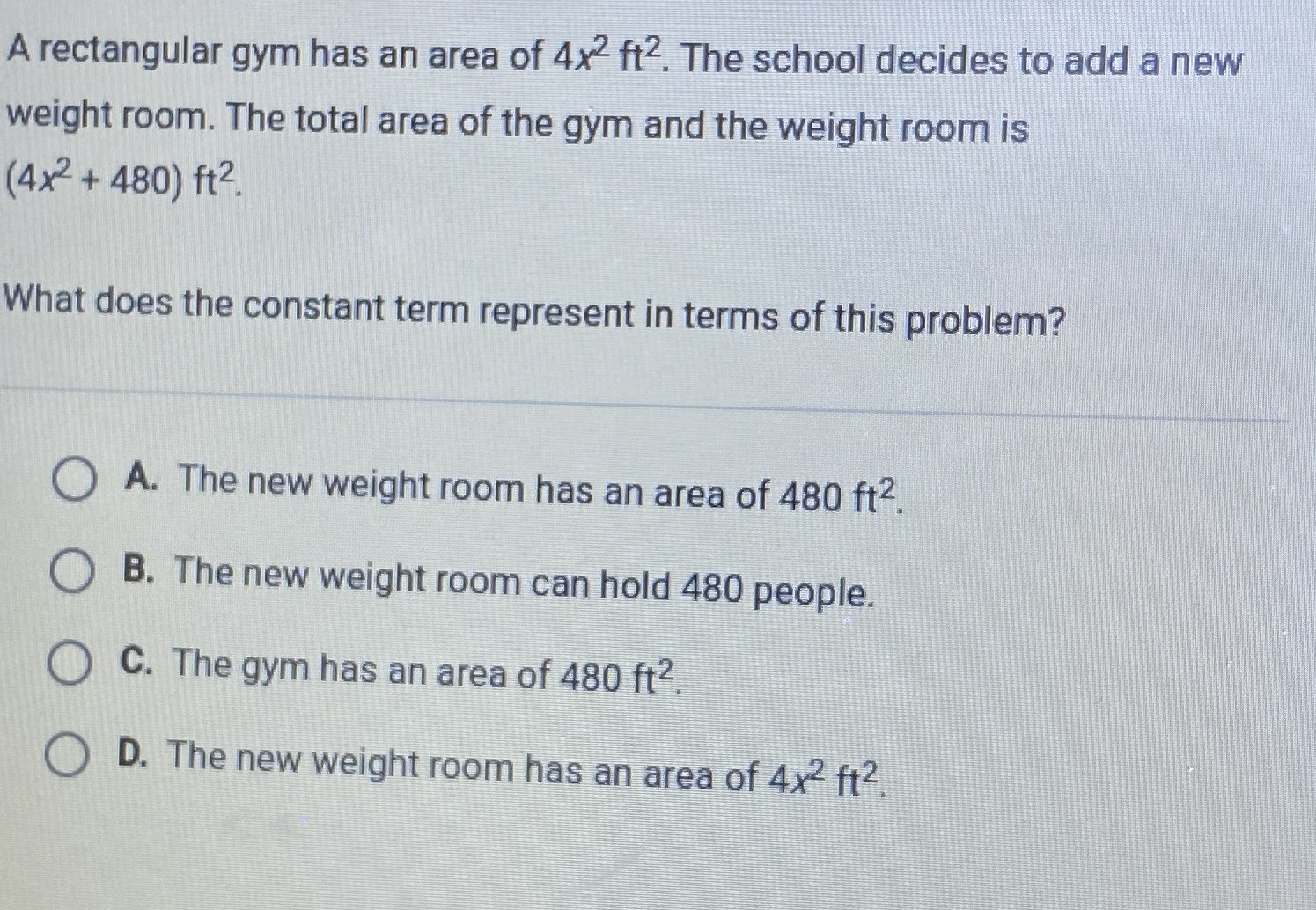### Still have math questions?

Algebra
QuestionA rectangular gym has an area of $$4 x ^ { 2 } ft ^ { 2 }$$ . The school decides to add a new weight room. The total area of the gym and the weight room is $$( 4 x ^ { 2 } + 480 ) ft ^ { 2 }$$ . What does the constant term represent in terms of this problem?

A.The new weight room has an area of $$480 ft^ { 2}$$

B. The new weight room can hold $$480$$ people.

C. The gym has an area of $$480 ft ^ { 2 }$$ .

D. The new weight room has an area of $$4 x ^ { 2 } ft ^ { 2 }$$ .